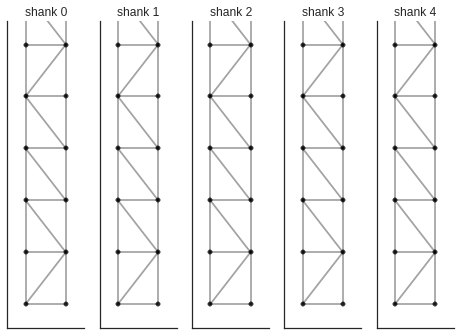# generating KlustaKwik graphs from the geometries¶

Justin Kiggins, May 2015 ([email protected] / @neuromusic)

Very large arrays make it unrealistic to manually define the probe architectures that SpikeDetekt2 expects. This example builds a channel_groups dictionary for a large array. It was inspired by the dense silicon arrays designed by Ed Boyden's group:5 shanks with 200 sites each and just 11 microns pitch between sites

### first, we need to be able to convert a geometry into a graph¶

we'll define a function which takes the geometry dict, extracts the coordinates, and uses the Delaunary transformation to generate a triangular tesselation. It then returns this graph in the form that SpikeDetekt2 expects

In :
from scipy import spatial
def get_graph_from_geometry(geometry):

# let's transform the geometry into lists of channel names and coordinates
chans,coords = zip(*[(ch,xy) for ch,xy in geometry.iteritems()])

# we'll perform the triangulation and extract the
tri = spatial.Delaunay(coords)

# then build the list of edges from the triangulation
indices, indptr = tri.vertex_neighbor_vertices
edges = []
for k in range(indices.shape-1):
for j in indptr[indices[k]:indices[k+1]]:
edges.append((chans[k],chans[j]))
return edges


### Now, we can build the probe definition from a few basic parameters¶

This is suitable to write directly to a *.prb file for SpikeDetekt2

In :
w = 2 # the width of the grid of sites
l = 100 # the length of the grid of sites
n_shanks = 5 # the number of shanks
pitch = 11 # site spacing in microns

channel_groups = {}
for sh in range(n_shanks):
# define the channels on this shank
channels = [sh*w*l+ch for ch in range(w*l)]

# get the physical coordinates by including the spacing
row_col = [(ch/w,ch%w) for ch in [c%(w*l) for c in channels]]
coords = [(pitch*c, pitch*(l-1)-pitch*r) for r,c in row_col]

# then  assign these to the geometry dictionary
geometry = {ch:xy for ch,xy in zip(channels,coords)}

# finally, use our function from above to define the graph
graph = get_graph_from_geometry(geometry)

channel_groups[sh] = {
'channels': channels,
'graph': graph,
'geometry': geometry,
}


### Did it work?¶

Let's plot it.

In :
%pylab inline
import seaborn as sns
sns.set_context(rc={'lines.markeredgewidth': 0.1})
sns.set_style('white')

f,ax = plt.subplots(1,n_shanks)
for sh in range(n_shanks):
coords = [xy for ch,xy in channel_groups[sh]['geometry'].iteritems()]

for pr in channel_groups[sh]['graph']:
points = [channel_groups[sh]['geometry'][p] for p in pr]
ax[sh].plot(*zip(*points),color='k',alpha=0.2)

ax[sh].set_xlim(-5,pitch*(w-1)+5)
ax[sh].set_ylim(-5,pitch*(w-1)*n_shanks+5)
ax[sh].set_xticks([])
ax[sh].set_yticks([])
ax[sh].set_title('shank %i'%sh)
ax[sh].scatter(*zip(*coords),color='0.2')
sns.despine()

Populating the interactive namespace from numpy and matplotlib